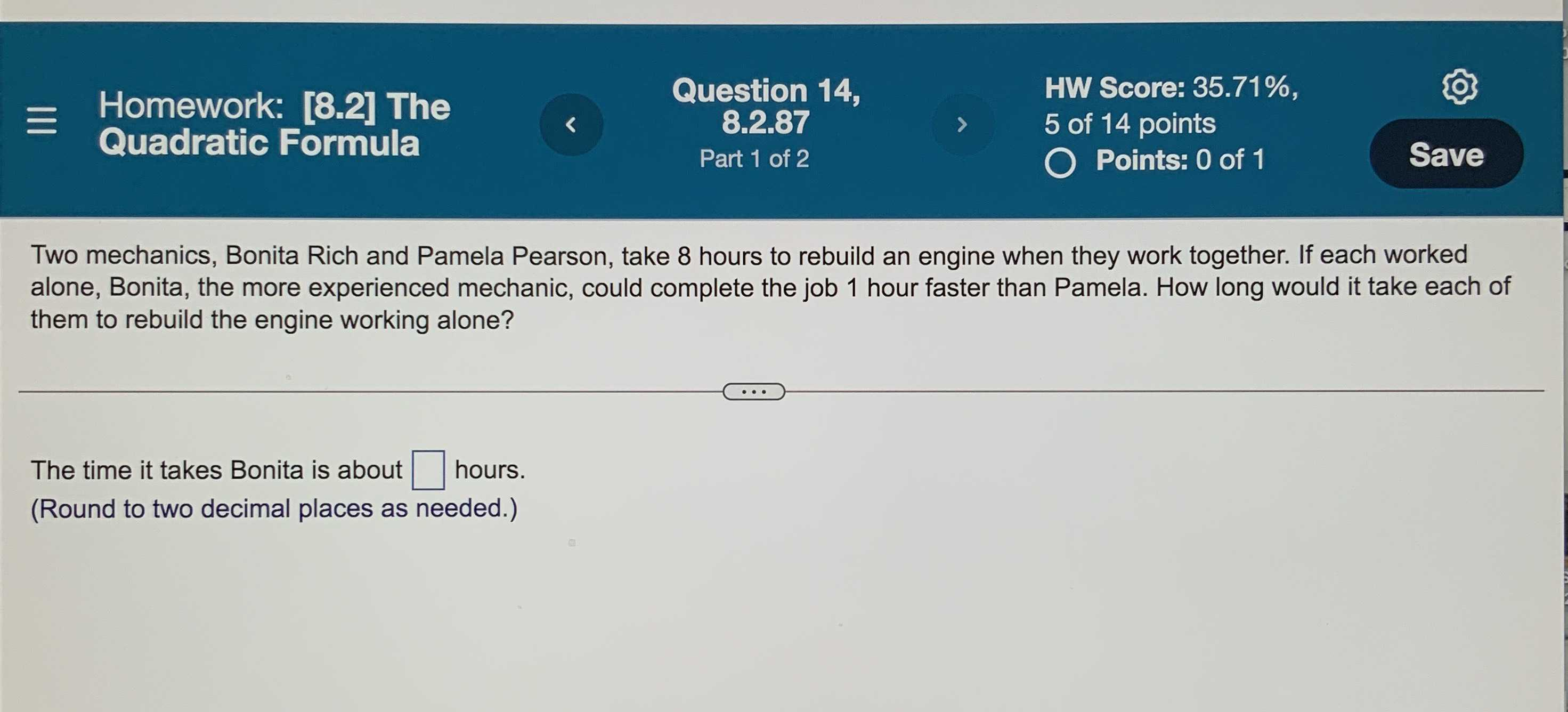### Still have math questions?

Algebra
QuestionTwo mechanics, Bonita Rich and Pamela Pearson, take $$8$$ hours to rebuild an engine when they work together. If each worked alone, Bonita, the more experienced mechanic, could complete the job $$1$$ hour faster than Pamela. How long would it take each of The time it takes Bonita is about $$\square$$ hours. them to rebuild the engine working alone?

The time it takes Bonita is about $$\square$$ hours.

(Round to two decimal places as needed.)